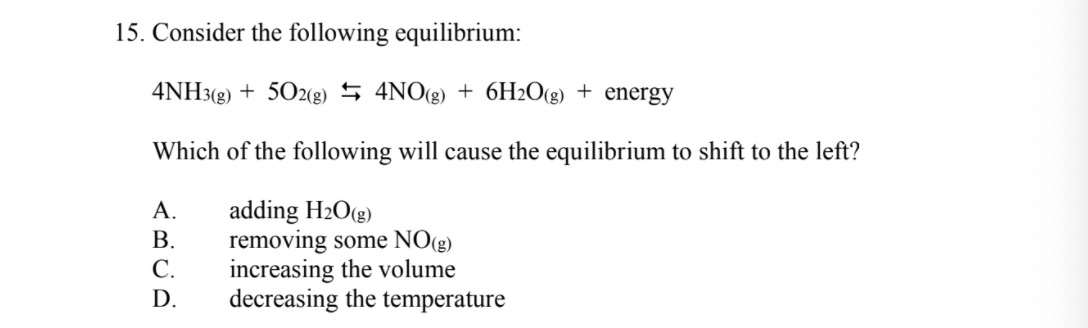# Question Solved1 Answer15. Consider the following equilibrium: 4NH3(g) + 502(g) \$ 4NO(g) + 6H2O(g) + energy Which of the following will cause the equilibrium to shift to the left? A. B. C. D. adding H2O(g) removing some NO(g) increasing the volume decreasing the temperatureTranscribed Image Text: 15. Consider the following equilibrium: 4NH3(g) + 502(g) \$ 4NO(g) + 6H2O(g) + energy Which of the following will cause the equilibrium to shift to the left? A. B. C. D. adding H2O(g) removing some NO(g) increasing the volume decreasing the temperature
More
Transcribed Image Text: 15. Consider the following equilibrium: 4NH3(g) + 502(g) \$ 4NO(g) + 6H2O(g) + energy Which of the following will cause the equilibrium to shift to the left? A. B. C. D. adding H2O(g) removing some NO(g) increasing the volume decreasing the temperature